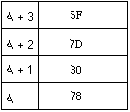# Corrections to Chapter 2 of Java Secrets, Primitive Data Types

p. 22: In both cases change "0000000000100001" to "0000000001000001"

p. 29: Change "8D01BA8D" to "4D01CA8D"

p. 32: The last three sentences should read:

Unlike the sign bit in integer data types, 1 represents a positive number and 0 represents a negative number. For example, 15.4 has sign 1, mantissa 154, and exponent 1. The number -0.7 × 10-32 has sign 0, mantissa 7, and exponent -33.

p. 33: Change "As discussed earlier in this chapter " to "As I mentioned earlier"

p. 34: 2**(24-1) should be written (2**24)-1

p. 34: In the section on finite precision, a lower case Greek letter pi should be used.

p. 41: Character 245 seems to have some sort of problem. Character 222 is supposed to be a capital thorn. Character 254 is supposed to be a lower case thorn. Character 168 is supposed to be a diaresis, not a vertical bar. Character 173 should be a hyphen. Character 174 should be a registered trademark sign. Character 175 is supposed no be a macron. Character 176 is supposed to be a degrees sign. Characters 178 and 179 are supposed to be superscripts. Character 180 is supposed to be an acute accent. Character 184 should be a cedilla. Character 185 is supposed to be a superscript one. Character 186 is supposed to be a masculine ordinal indicator. Character 192 is supposed to a be a capital A with grave. Pretty much all characters from 192 through 255 is incorrect, apparently due to poor typesetting. This entire chart needs to be redone from my originals.

p. 46: In the second to last paragraph on p. 46, change "false." to "false". In other words move the period outside the quotation marks (and yes, I know this violates standard American English grammar.)

p. 51: 7D and 6F are swapped in Figure 2-3. The correct figure is belowp. 52: change "11111111 is not 255 but rather -128" to "11111111 is not 255 but rather -1".

p. 53: change "then if it's written" to "and if it's written" at the end of the second paragraph

p. 54: Change the second paragraph to the following:

The second place in which conversion of primitive types can take place is in binary arithmetic expressions like a + b or 7/0.65. The expression is evaluated using the widest type given as an argument to the binary operator, where doubles are wider than floats which are wider than longs which are wider than ints. Thus if any of the operands are doubles, all operands are promoted to doubles. If no operands are doubles but some are floats, then all operands are promoted to floats. If no operands are floats or doubles but some are longs, then all operands are promoted to longs. Finally if an expression contains no floats, doubles, or longs, then all operands are promoted to ints. All arithmetic in Java uses at least ints. Shorts, bytes, and chars are never used directly in arithmetic expressions.
p. 55: remove the hyphen from 000000000000-00000000000001011100

p. 57: change "double is converted to a double" to "double is converted to an int" in the thrid to last paragraph

p. 58: "the byte value 37" should be the "the byte value 21"

p. 59: The sentence, "The result, shown in the bottom row, is 01001100, that is, 76" should read "The result, shown in the bottom row, is 01001000, that is 72." Figure 2-5 should also be changed to say "=72". and the figure should also have 78 in the top left hand cell and -23 in the cell below that.

p. 61: Figure 2-6 should have 78 in the top left hand cell and -23 in the cell below that.

p. 62: Change "The result shown in the bottom row is 10100111-89" to "The result shown in the bottom row is 10100111 or -89 in decimal."

In Figure 2-7 "=-17" should be "=-89". In other words that table should look like this:

 78 0 1 0 0 1 1 1 0 -23 1 1 1 0 1 0 0 1 78 ^ -23 = -89 1 0 1 0 0 1 1 1

p. 67: Each line of Table 2-9 and Table 2-10 is missing one `>`. In other words, those tables should look something like this

 -23 11111111111111111111111111101001 -23 >> 1 = -12 11111111111111111111111111110100 -23 >> 2 = -6 11111111111111111111111111111010 -23 >> 3 = -3 11111111111111111111111111111101 -23 >> 4 = -2 11111111111111111111111111111110 -23 >> 5 = -1 11111111111111111111111111111111 -23 >> 6 = -1 11111111111111111111111111111111
 -23 11111111111111111111111111101001 -23 >>> 1 = 2,147,483,636 01111111111111111111111111110100 -23 >>> 2 = 1,073,741,818 00111111111111111111111111111010 -23 >>> 3 = 536,870,909 00011111111111111111111111111101 -23 >>> 4 = 268,435,454 00001111111111111111111111111110 -23 >>> 5 = 134,217,727 00000111111111111111111111111111 -23 >>> 6 = 67,108,863 00000011111111111111111111111111
p. 68: The algorithm for converting little-endian to big-endian fails when any byte has its high order bit set, because the implicit conversion to int when using the `<<` operator throws in a sign bit. In addition, just because four bytes aren't read doesn't necessarily mean the end of stream has been reached. A much better, correct algorithm is:

``````  public static int readLittleEndianInt(InputStream in) throws IOException {

int byte1, byte2, byte3, byte4;

int byte1 = in.read();
int byte2 = in.read();
int byte3 = in.read();
int byte4 = in.read();

if (byte4 == -1) throw new EOFException();

return  (byte4 << 24)
|  (byte3 << 24) >>>  8)
|  (bytte2 << 24) >>> 16)
|  (byte1 << 24) >>> 24 );

}``````

Copyright 1997, 1998, 2002 Elliotte Rusty Harold
elharo@metalab.unc.edu